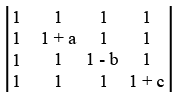# ISRO Scientist or Engineer Mechanical 2009

Instructions

For the following questions answer them individually

Question 71

# The rake angle of a cutting tool is $$15^\circ$$, shear angle $$45^\circ$$ and cutting velocity 35 m/min. Whatis the velocity of chip along the tool face?Question 72

# The value of the determinant given below isQuestion 73

# The locus of the point Z satisfying the condition $$arg \frac{z - 1}{z + 1} = \frac {\pi}{3}$$ isQuestion 74

# In a simple micrometer with screw pitch 0.5 mm and divisions on thimble 50, the reading corresponding to 5 divisions on barrel and 12 divisions on thimble isQuestion 75

# It is required to cut screw threads of 2mm pitch on a lathe. The lead screw has a pitch of 6 mm. If the spindle speed is 60 rpm, then the speed of the lead screw will beQuestion 76

# If $$u = \log(\tan x + \tan y),$$ then $$\sin 2x \frac{\partial u}{\partial x} + \sin 2y \frac{\partial u}{\partial y}$$ isQuestion 77

# The value of $$\lambda$$ for which the equations $$2 \lambda x - 3y + \lambda - 3 = 0, 3x - 2y + 1 = 0$$ and $$4x - \lambda y + 2 = 0$$ are consistent isQuestion 78

# If $$\overline{a} + \overline{b} + \overline{c} = 0$$ and $$|\overline{a}| = 3, |\overline{b}| = 5, |\overline{c}| = 7,$$ then the angle between $$\overline{a}$$ and $$\overline{b}$$ isQuestion 79

# The image of the point P(1,3,4) in the plane 2x - y + z = -3 isQuestion 80

# Find a particular integral of the differential equation $$(D^2 - 4D + 3)y = \sin 3x \cos 2x$$OR